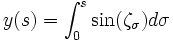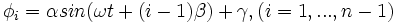# Robot Snake

Snake Robot

## Team Members

• Michael Hwang - Electrical Engineer - Class 2008
• Andrew Long - Mechanical Engineer - Class 2009
• Clara Smart - Electrical Engineer - Class 2009

## Snake Motion

Snakes are able to adapt their movement to various environments. For instance, snakes can move across extreme environments such as sand, mud and water. Research has discovered there are four types of snake motion, as shown in the image. These motions include; serpentine movement, rectilinear movement, concertina movement and side-winding movement.<ref>Ma, Shugen. "Analysis of creeping locomotion of a snake-like robot." Advanced Robotics Vol.15, No.2 (2001): 205</ref> The most common motion exhibited by most snakes is serpentine motion where section follows a similar path. In order for snakes to successfully locomote using serpentine motion, the belly of the snake must have anisotropic coefficient of friction for the normal and tangential directions. Specifically, the normal friction must be greater than the tangential friction. As a result, when the snake exhibits a force on the ground, it will move in the tangential direction without slipping in the normal direction.<ref>Saito, Fukaya, Iwasaki. "Serpentine Locomotion with Robotic Snakes". IEEE Control Systems Magazine (Feb 2002): 66.<ref/>

Many robots are limited by the use of motorized wheels. However, there are many advantages for building a robot that mimics the motion of a snake. Several advantages for movement of snake robot are listed below:

• Move across uneven terrain since it is not dependent on wheels
• Possibly swim if water-proofed
• Move across soft ground such as sand since it can distribute its weight across wider area

Also, from a systems standpoint, the snake robot can be very modular with many redundant segments. As a result, it is very easy to replace broken segments as well as shorten or lengthen the robot.

Although there are many advantages for building a snake like robot, there are several disadvantages which are listed below:

• Low power and movement efficiency
• High cost of actuators (servos or motors)
• Difficult to control high number of degrees of freedom

Cite = Page 206

## Robot Snake Motion

Real snake motion does not follow specified equations. However, research has proven that the serpentine motion of a snake can be modeled with the following equations:$x(s)= \int_{0}^{s} \cos (\zeta_\sigma) d\sigma$$y(s)= \int_{0}^{s} \sin (\zeta_\sigma) d\sigma$

ζσ = acos(bσ) + cσ

where the parameters a, b, and c determine the shape of the serpentine motion. The graph shows how the parameters influence the serpentine curve. Basically, a changes the appearance of the curve, b changes the number of phases, and c changes the direction.

The serpentine curve can be modeled with a snake like robot by changing the relative angles between the snake robot segments using the following formula with the number of segments (n):$\phi_i = \alpha sin(\omega t +(i-1)\beta ) + \gamma, \left ( i=1, ..., n-1 \right )$

where α , β , and γ are parameters used to characterize the serpentine curve and are dependent on a, b, and c as shown below:$\alpha = a \left | \sin \left ( \frac{\beta}{2} \right ) \right |$$\beta = \frac{b}{n}$$\gamma = -\frac{c}{n}$

The equations above for φi,α,β, and γ were used in this snake like robot as shown in the code section.

## Mechanical Design

As discussed above, real snakes move with anisotropic coefficients of friction. It is difficult to locate materials with this property, but passive wheels satisfy the requirements for differences in friction. The friction will be lower in the direction of rolling, thus providing the required difference in friction. The only problem with this approach is that the wheel may slide in the normal direction if the weight applied to the wheel is not sufficient.

Needs Description of Chassis + Picture + Template

Needs Description of wheels (pulley + oring) + Picture

Needs Description of stand-offs

Needs Description of housing (pipe) say it is temporary

## Electronics

Needs description of servo - include discussion of how we aligned them (through code)

Needs circuit diagram for servo boards and/or picture

Needs description of ribbon cable? (ie be careful with it)

Needs reason for terminator

## PIC Code

Obviously needs code with some explanation of how we used it for multiple servos

Needs to discuss wireless communication via Xbee and link to Xbee site

## Results

Initial Design Discussion? May not be necessary

Discuss how it went well